# 8+ Multiplication Worksheets Examples in PDF

Multiplication is one of the basic math operations that we using in almost every aspect of our everyday living. You might notice it or you may not be too conscious about it but you are already multiplying things such as when you would buy something at the grocery or convenience store or when you would be computing your daily expenses at the end of the day. And with that, it is recommended that we master the art of multiplying numbers as early as in our childhood. This is to train our minds in multiplying varying values of numbers quickly and since it is one of the four basic math operations, it can be helpful in our day-to-day living.

## Multiplication Worksheet Example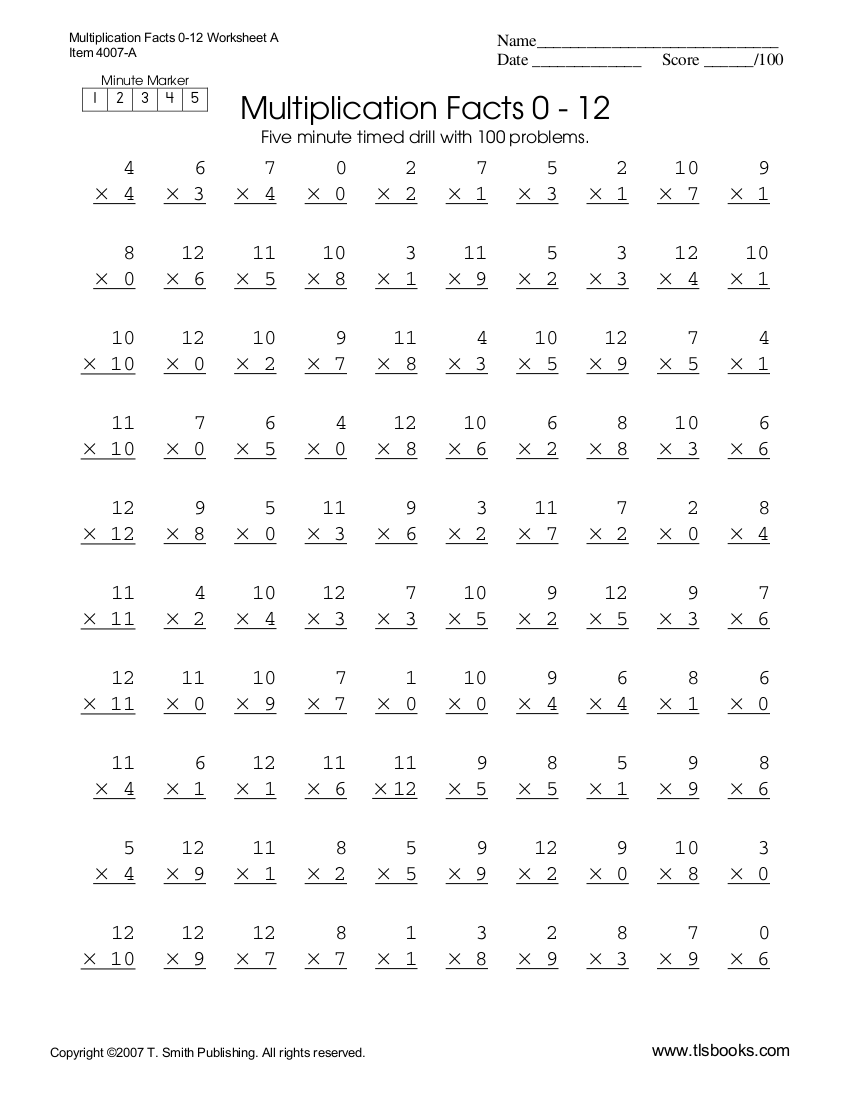tlsbooks.com

## Double Digit Multiplication Worksheet Example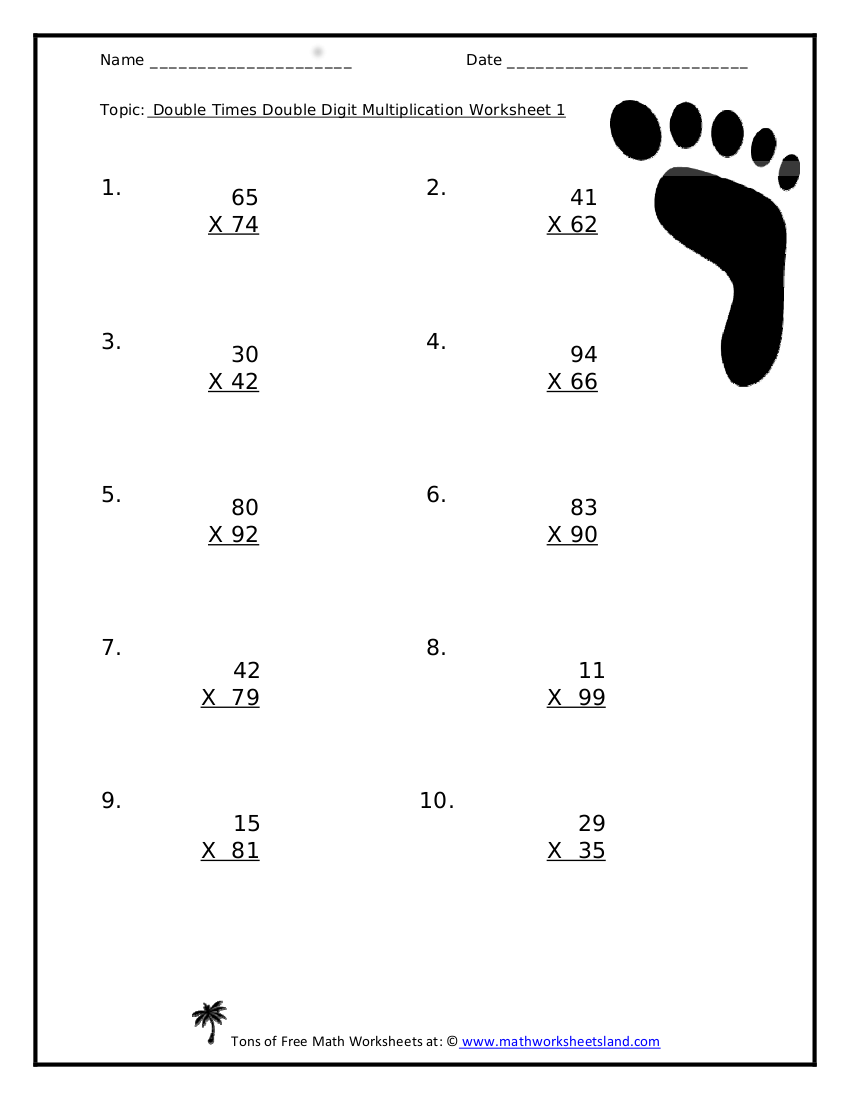mathworksheetsland.com

## Multiplication Drills Worksheet Example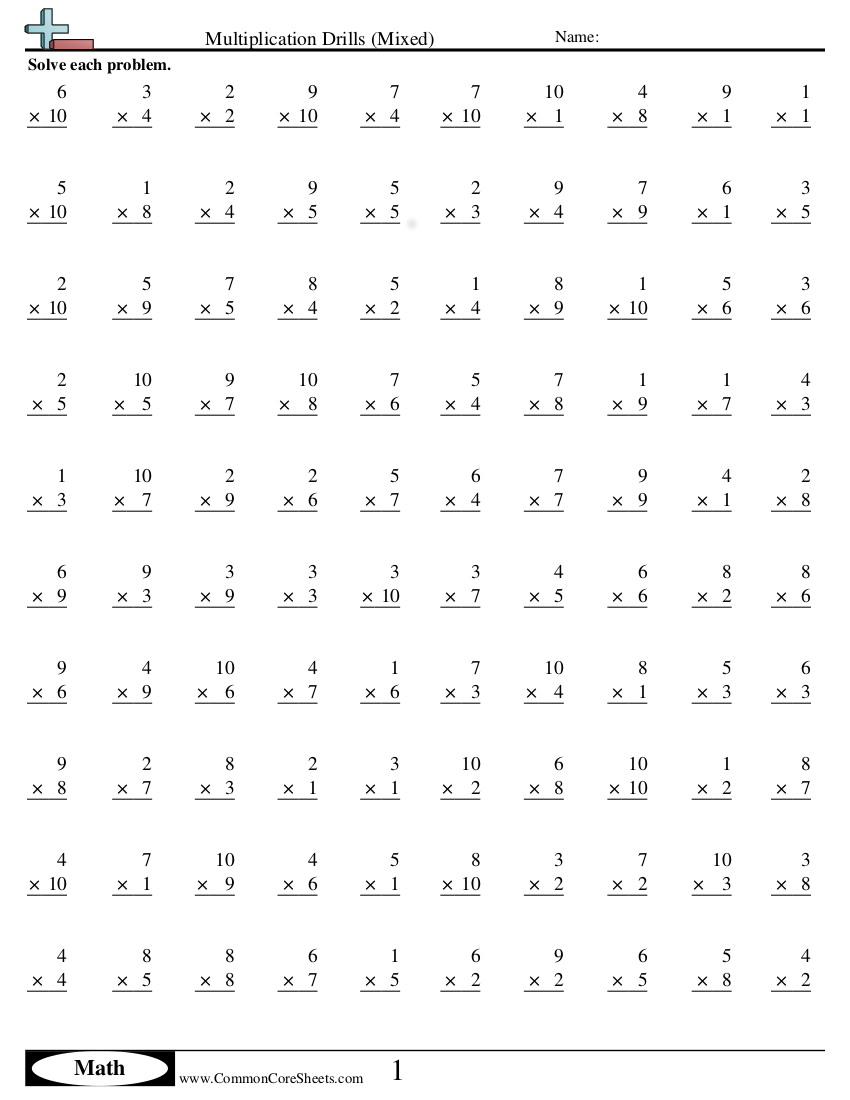commoncoresheets.com

## Multiplication Worksheets Example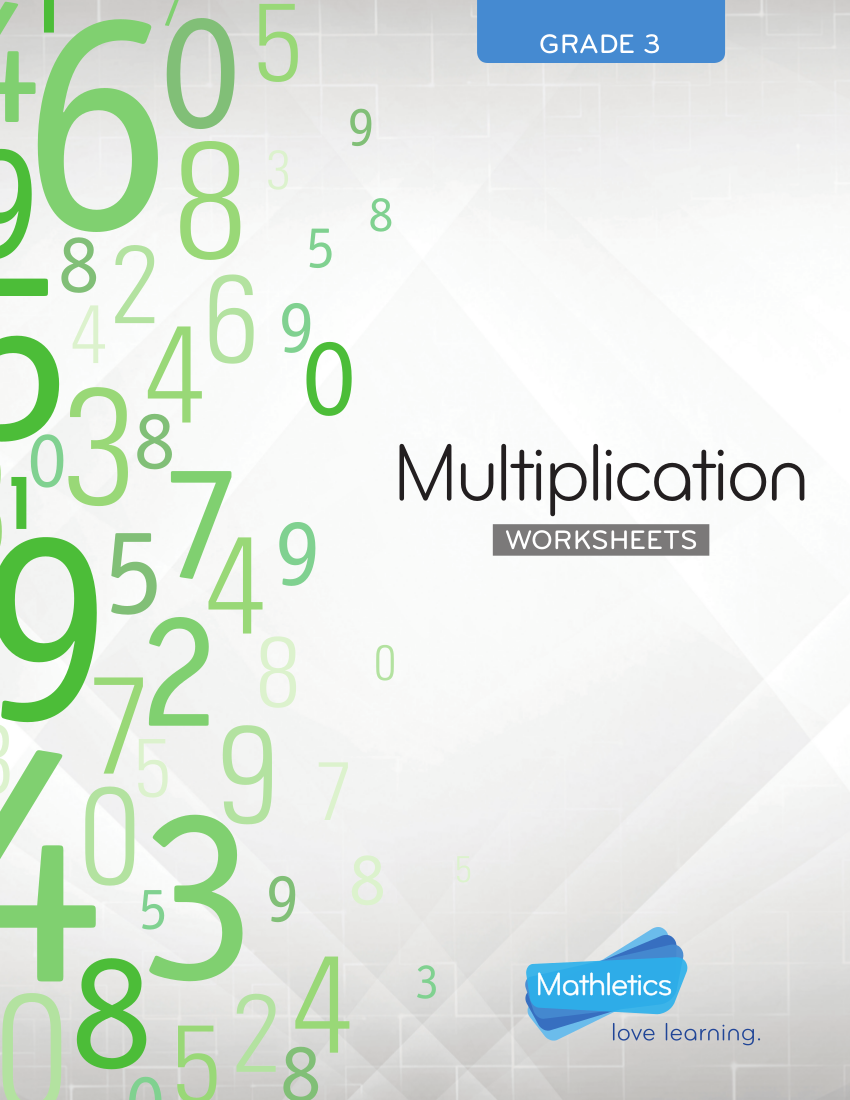3plearning.com

## Multiplication Fast Facts

• You might wonder why multiplication is often described as repeated addition. This is because if you would get the product of 4 x 4, it is equal to the sum of four fours which goes like this: 4 + 4 + 4 + 4. You may also see time worksheets examples in pdf.
• In order to determine whether an equation is a multiplication, you have to look at the sign used. Multiplication is actually symbolized in three ways:
• “x” as in 4 “x” 4 = 16
• a centered dot ( · ) as in 4 · 4 = 16
• writing the numbers next to each other with the use of parentheses () as in 4(4) = 16 or (4)(4) = 16
• Keep in mind that the factor is the number that is being used as a multiplier in an equation, the multiplier is the one or two numbers that are being combined in the act of multiplying both in order to come up with a product, and the product is the result after multiplying two or more numbers. You may also check out expense worksheet examples in pdf.
• The answer to a multiplication problem or equation is called as the product and it can be derived by multiplying two multipliers. For examples, in the equation 4 x 4 = 16, the product here is 16 and the multipliers, which can also be called as the factors or terms, is 4 x 4. You might be interested in reading comprehension worksheet examples in pdf.
• When multiplying with decimal numbers, you might get overwhelmed but do not because all you have to do is just multiply the decimal fractions like natural numbers but just keep in mind to place the decimal point in the product according to the total number of decimal places of both multipliers.
For example:
4.44 x 4.44 = 19.7136.
Since there is a total of four decimal places from both of the multipliers, you have then to make sure that you have four decimal places on the product. You may also like resume worksheet examples in pdf.
• Ever encountered multiplication equation wherein the multipliers had varying signs? Do not worry; just multiply the numbers as if the signs do not exist. The rules go the product goes:
• If the two multipliers or factors has the same sign, give the product the similar sign. Hence, if it’s both negative, the product is also negative and if both are positive, then the product should also be positive. So, for example, 4 x 4 = 16 since both factors are positive, and -4 x -4 = -16 since both factors are negative.
• If the two multipliers have varying signs, the product should automatically use a negative sign. So, for example, 4 x -4 = -16.

## Quick Multiplication Drill Worksheet Example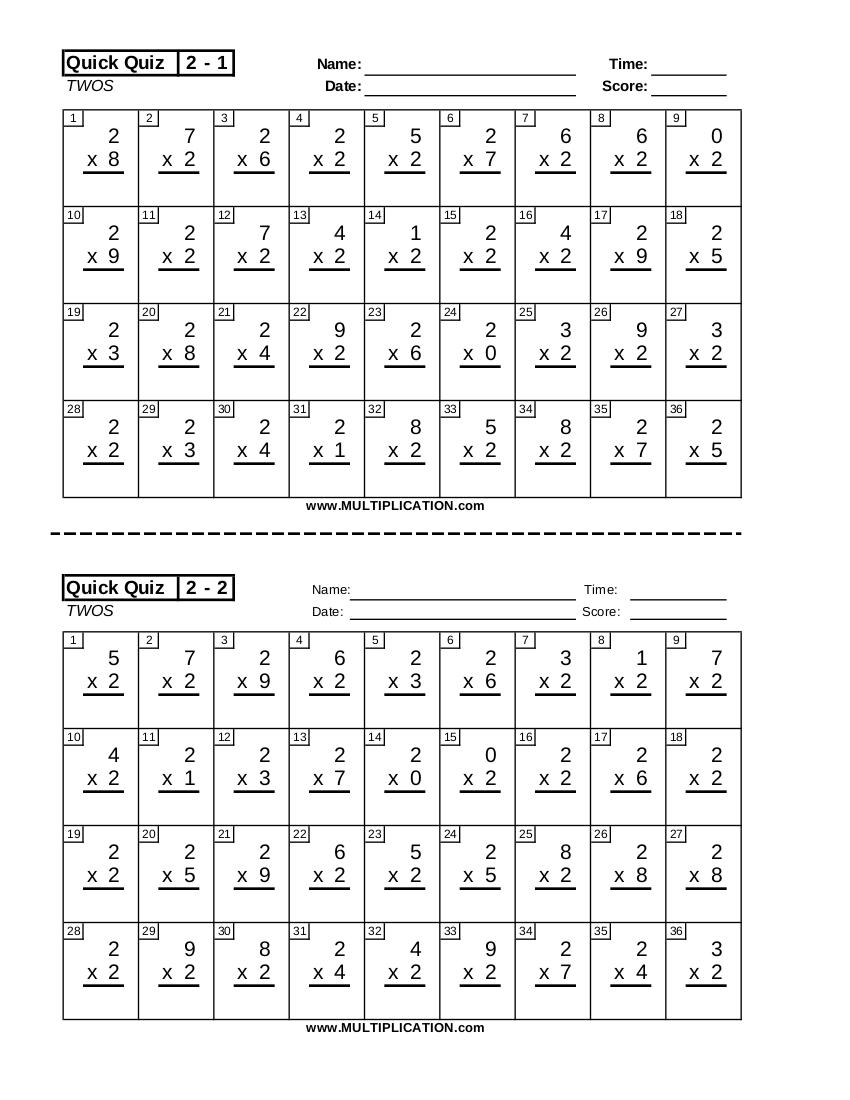multiplication.com

## Two Digit Multiplication Worksheet Example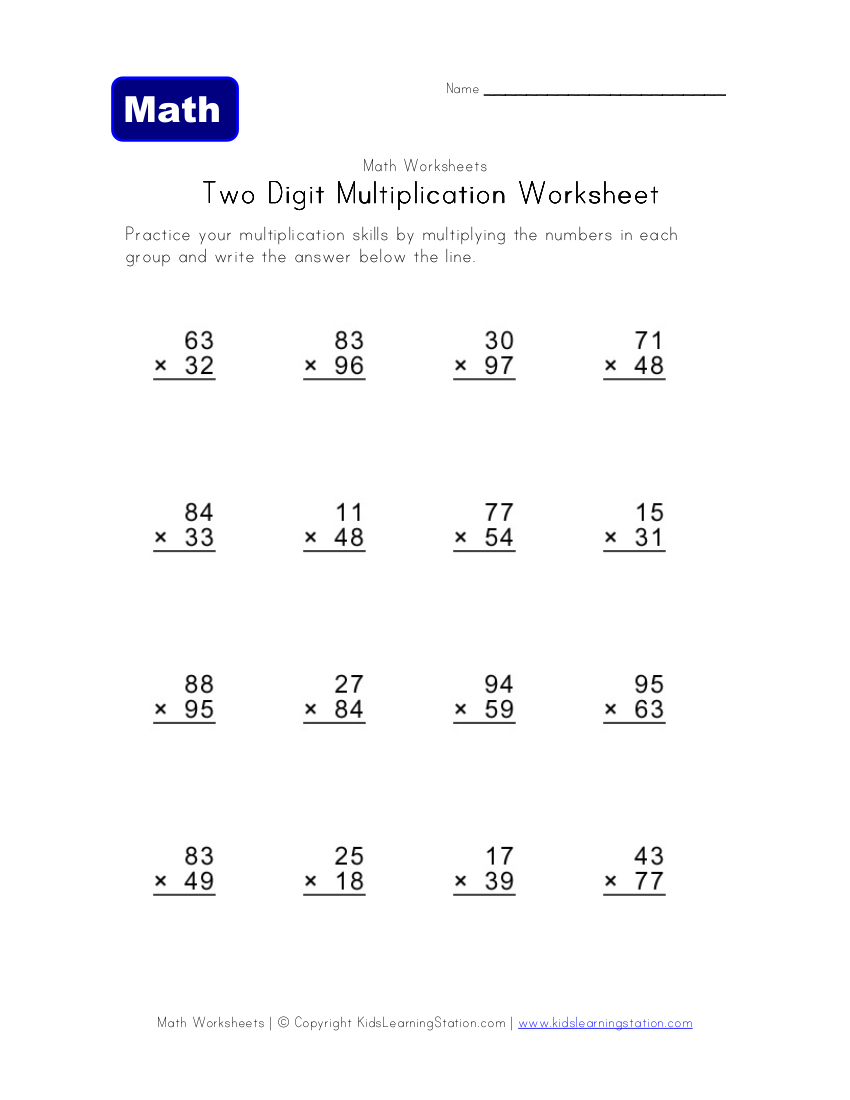triad.k12.oh.u

## Multiplication Squares Worksheet Example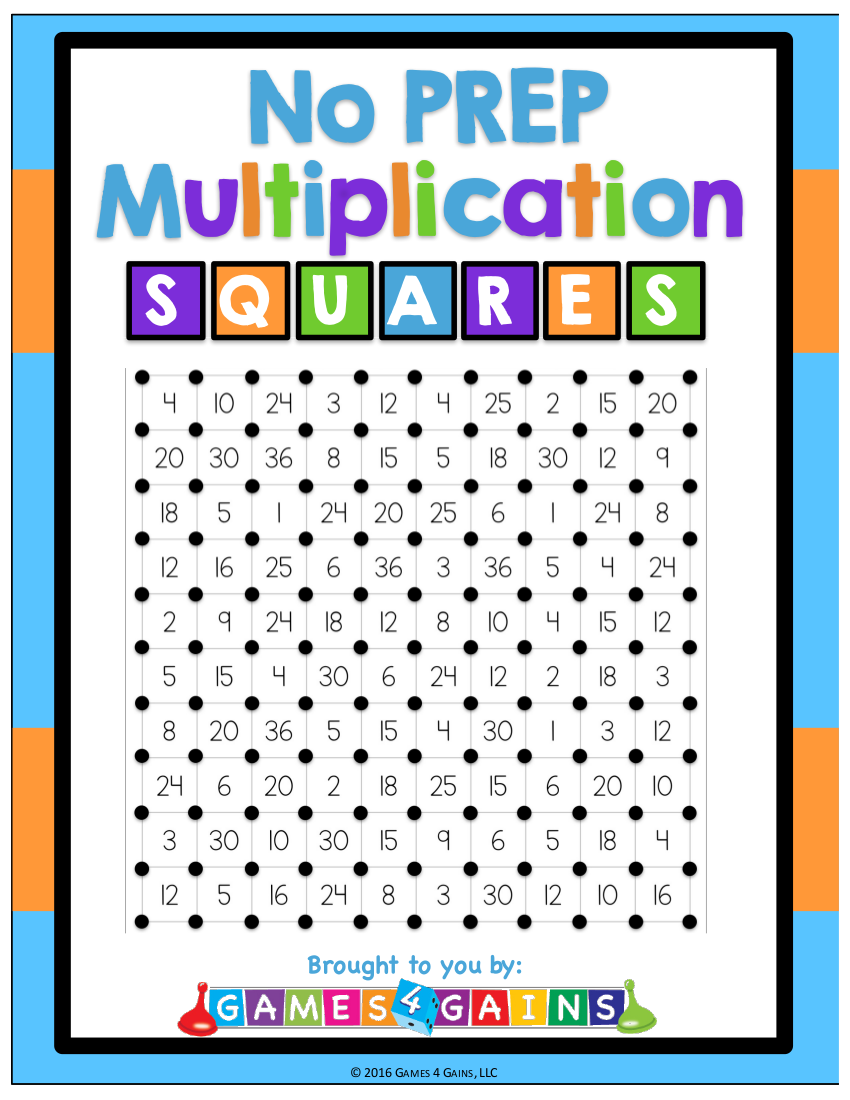pasco.k12.fl.us

## Short Multiplication Worksheet Example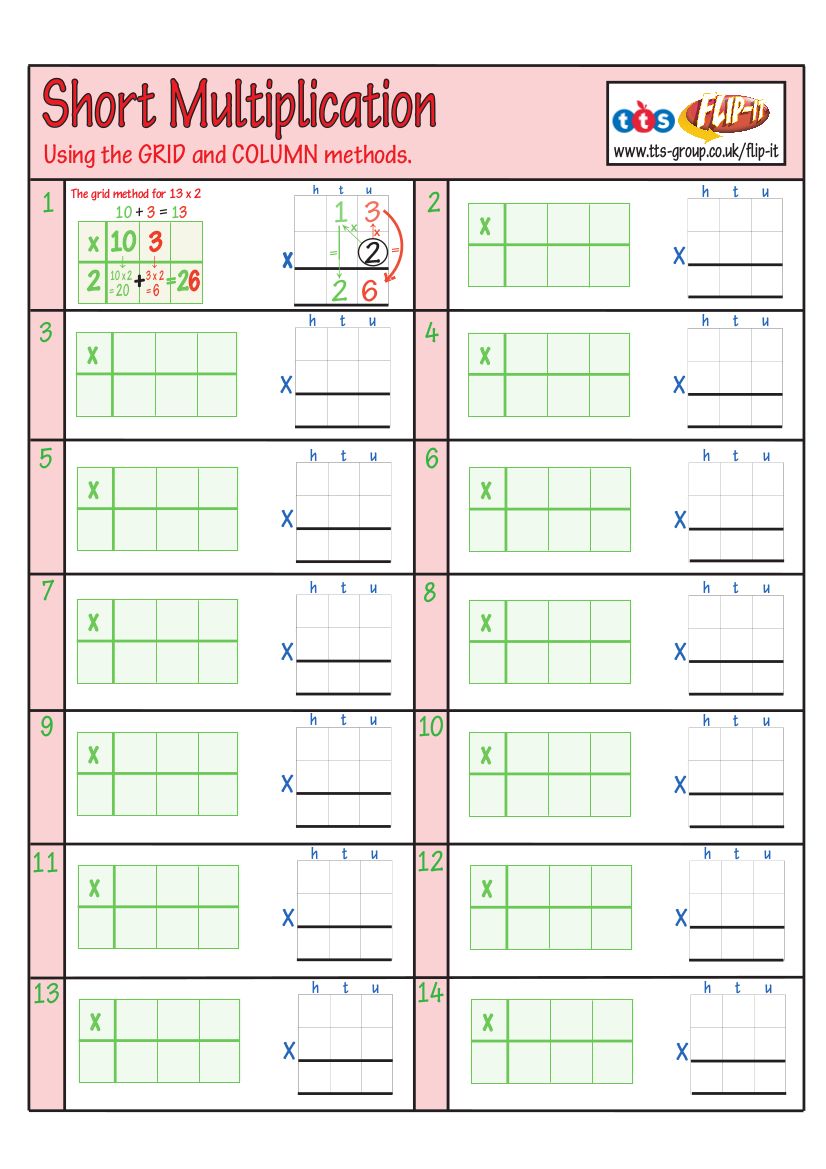tts-group.co.uk

Even if multiplication seems like the most ordinary thing in the world of mathematics, there are still some interesting facts about it. You may see some of it in the following:

• The word “multiply” is derived from the Latin term multus which means “multi” and the Latin term plex which means“fold”. You may also like self-assessment worksheet examples in pdf.
• Multiplication is just another method to repeatedly add numbers, so it is safe to call it “repeated addition”. You may also see writing worksheet examples in pdf.
• One of the properties in multiplication is the zero (0) property. The zero property means that whenever you multiply zero by any number (including zero), the answer is always zero. You may also see activity sheet examples & samples.

• Multiplication is actually a type of arithmetic and did you know that arithmetic is the oldest and the most basic branch of mathematics? You may also see sheet examples in DOC.

• Did you know that multiplication has an inverted version? It’s Division. How? Take this for example: 4 x 4 = 16. Now, if you would inverse it using division, it goes like this: 16 / 4 = 4. You may also check out coaching worksheet examples in pdf.

• When you would be multiplying an even number with the number, the answer or the product also ends up with an even number. Take this for example:
6 x 1 = 6, 6 x 2 = 12, 6 x 3 = 18, 6 x 4 = 24, 6 x 5 = 32, 6 x 6 = 36, 6 x 7 = 42, 6 x 8 = 48, 6 x 9 = 54, and 6 x 10 = 60

• Did you know that the multipliers of an equation is previously referred to as multiplier and multiplicand? But now, the two is just referred to as multipliers and sometimes both called as factors. You may also check out math worksheets for students.
• The multiplication tables are also referred to as the “Table of Pythagoras.” It is coined in honor of Pythagoras of Samos, who is a famous Ionian Greek philosopher and mathematician. You may also like grammar worksheets.
• There was a clergyman way back in the 1500s who first used the symbol “?” for multiplication instead of what we know now as “x” symbol used for multiplying numbers. He was William Oughtred and he offered free math lessons during his time. However, Gottfried Wilhelm Leibniz objected this, thinking that it resembled the unknown “x”. You may also like biography worksheet examples.
• The Babylonians were the first to use multiplication tables and that happened over 4,000 years ago! You might be interested in goal setting worksheets.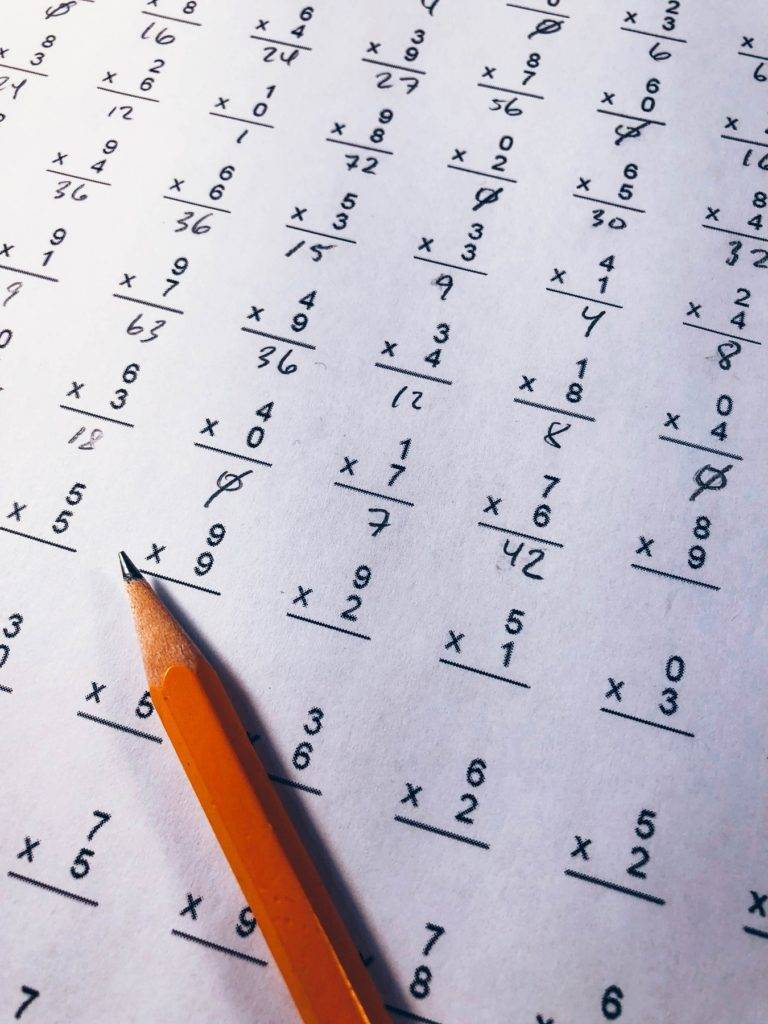## Quotes and Sayings Inspired by Multiplication

We all know that multiplication is used in our daily lives, so do not be surprised to read the following quotations and famous sayings made by famous people that are inspired by the concept of multiplication. After all, mathematics is a part of our lives:

• “Civilization is the limitless multiplication of unnecessary necessities.”
? Mark Twain
? Israelmore Ayivor
• “In moments of crisis, all you gotta do is review your multiplication tables, and it’ll all blow over!”
? Andrea Camilleri
• “But I was thinking of a way To multiply by ten, And always, in the answer, get The question back again.”
? Lewis Carroll
• “Man falls from the pursuit of the ideal of plain living and high thinking the moment he wants to multiply his daily wants. Man’s happiness really lies in contentment.”
? Mahatma Gandhi
• “I am for a government rigorously frugal & simple, applying all the possible savings of the public revenue to the discharge of the national debt; and not for a multiplication of officers & salaries merely to make partisans, & for increasing, by every device, the public debt, on the principle of being a public blessing.”
? Thomas Jefferson
• “Thus you may multiply each stone 4 times & no more for they will then become oyles shining in ye dark and fit for magical uses. You may ferment them with gold and silver, by keeping the stone and metal in fusion together for a day, & then project upon metalls. This is the multiplication of ye stone in virtue. To multiply it in weight ad to it of ye first Gold whether philosophic or vulgar.”
? Isaac Newton
• “If it is a terrifying thought that life is at the mercy of the multiplication of these minute bodies [microbes], it is a consoling hope that Science will not always remain powerless before such enemies.”
? Louis Pasteur
• “A society’s competitive advantage will come not from how well its schools teach the multiplication and periodic tables, but from how well they stimulate imagination and creativity.”
? Albert Einstein

Have fun solving these multiplication worksheets! You may also see Math Worksheets for Students.